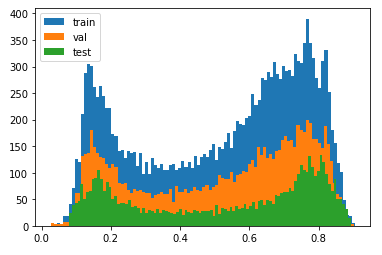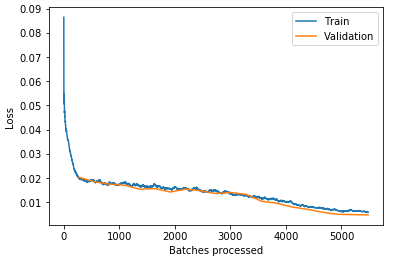# Issues in predictions when validation results are good

Hi, I have a tabular data-size `(32147, 31)`

My y_var is continuous variable ranges from 0 to 1. I split the data to train and test and set the validation index in the `TabularList`.
This is the model setting:

``````learn = tabular_learner(data, layers=[750,500], ps=[0.01,0.1], emb_drop=0.04,
y_range=y_range, metrics=rmse)
``````

Here you can see the distribution of the train, validation, and test y_var:As you can see, the test set is well represented in the train set.

My losses plot also looks good:In my field, 0.01 MSE (rmse=0.07) is very good. So I’m happy with this result.
However, when I predict on the test set, I get much worse:

This is how I make my predictions:
`y_pred = [learn.predict(df_test.iloc[i])[-1].numpy() for i in range(df_test.shape)]`

I know it is not the fastest way to predict but couldn’t find another way to do that.

What can cause good results in the training and bad ones in the predictions? The first thing that comes to mind is overfitting, but I don’t think this is the case here because the test set seems to be well represented in the train test.
Any suggestions on how I can improve my predictions?